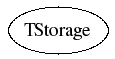library: libCore#include "TStorage.h"

# TStorage

class description - header file - source file

## class TStorage### Function Members (Methods)

Display options:
Show inherited
Show non-public
public:
 TStorage() TStorage(const TStorage&) virtual ~TStorage() static void AddToHeap(ULong_t begin, ULong_t end) static void* Alloc(size_t size) static TClass* Class() static void Dealloc(void* ptr) static void EnableStatistics(int size = -1, int ix = -1) static void EnterStat(size_t size, void* p) static FreeHookFun_t GetFreeHook() static void* GetFreeHookData() static ULong_t GetHeapBegin() static ULong_t GetHeapEnd() static size_t GetMaxBlockSize() static Bool_t HasCustomNewDelete() virtual TClass* IsA() const static Bool_t IsOnHeap(void* p) static void* ObjectAlloc(size_t size) static void* ObjectAlloc(size_t size, void* vp) static void ObjectDealloc(void* vp) static void ObjectDealloc(void* vp, void* ptr) TStorage& operator=(const TStorage&) static void PrintStatistics() static void* ReAlloc(void* vp, size_t size) static void* ReAlloc(void* vp, size_t size, size_t oldsize) static char* ReAllocChar(char* vp, size_t size, size_t oldsize) static Int_t* ReAllocInt(Int_t* vp, size_t size, size_t oldsize) static void RemoveStat(void* p) static void SetCustomNewDelete() static void SetFreeHook(FreeHookFun_t func, void* data) static void SetMaxBlockSize(size_t size) static void SetReAllocHooks(ReAllocFun_t func1, ReAllocCFun_t func2) virtual void ShowMembers(TMemberInspector& insp, char* parent) virtual void Streamer(TBuffer& b) void StreamerNVirtual(TBuffer& b)

### Data Members

private:
 static ULong_t fgHeapBegin begin address of heap static ULong_t fgHeapEnd end address of heap static size_t fgMaxBlockSize largest block allocated static FreeHookFun_t fgFreeHook function called on free static void* fgFreeHookData data used by this function static ReAllocFun_t fgReAllocHook custom ReAlloc static ReAllocCFun_t fgReAllocCHook custom ReAlloc with length check static Bool_t fgHasCustomNewDelete true if using ROOT's new/delete

## Class Description

```
TStorage

Storage manager. The storage manager works best in conjunction with
the custom ROOT new and delete operators defined in the file
NewDelete.cxx (libNew.so). Only when using the custom allocation
operators will memory usage statistics be gathered using the
TStorage EnterStat(), RemoveStat(), etc. functions.
Memory checking is by default enabled (when using libNew.so) and
usage statistics is gathered. Using the resource (in .rootrc):
Root.MemStat one can toggle statistics gathering on or off. More
specifically on can trap the allocation of a block of memory of a
certain size. This can be specified using the resource:
Root.MemStat.size, using the resource Root.MemStat.cnt one can
specify after how many allocations of this size the trap should
occur.
Set the compile option R__NOSTATS to de-activate all memory checking
and statistics gathering in the system.

```
void EnterStat(size_t size, void *p)
``` Register a memory allocation operation. If desired one can trap an
allocation of a certain size in case one tries to find a memory
leak of that particular size. This function is only called via
the ROOT custom new operators.
```
void RemoveStat(void *vp)
``` Register a memory free operation. This function is only called via
the custom ROOT delete operator.
```
void * Alloc(size_t size)
``` Allocate a block of memory, that later can be resized using
TStorage::ReAlloc().
```
void Dealloc(void *ptr)
``` De-allocate block of memory, that was allocated via TStorage::Alloc().
```
void * ReAlloc(void *ovp, size_t size)
``` Reallocate (i.e. resize) block of memory.
```
void * ReAlloc(void *ovp, size_t size, size_t oldsize)
``` Reallocate (i.e. resize) block of memory. Checks if current size is
equal to oldsize. If not memory was overwritten.
```
char * ReAllocChar(char *ovp, size_t size, size_t oldsize)
``` Reallocate (i.e. resize) array of chars. Size and oldsize are
in number of chars.
```
Int_t * ReAllocInt(Int_t *ovp, size_t size, size_t oldsize)
``` Reallocate (i.e. resize) array of integers. Size and oldsize are
number of integers (not number of bytes).
```
void * ObjectAlloc(size_t sz)
``` Used to allocate a TObject on the heap (via TObject::operator new()).
Directly after this routine one can call (in the TObject ctor)
TStorage::IsOnHeap() to find out if the just created object is on
the heap.
```
void * ObjectAlloc(size_t , void *vp)
``` Used to allocate a TObject on the heap (via TObject::operator new(size_t,void*))
in position vp. vp is already allocated (maybe on heap, maybe on
stack) so just return.
```
void ObjectDealloc(void *vp)
``` Used to deallocate a TObject on the heap (via TObject::operator delete()).
```
void ObjectDealloc(void *vp, void *ptr)
``` Used to deallocate a TObject on the heap (via TObject::operator delete(void*,void*)).
```
void SetFreeHook(FreeHookFun_t fh, void *data)
``` Set a free handler.
```
void SetReAllocHooks(ReAllocFun_t rh1, ReAllocCFun_t rh2)
``` Set a custom ReAlloc handlers. This function is typically
called via a static object in the ROOT libNew.so shared library.
```
void PrintStatistics()
``` Print memory usage statistics.
```
void EnableStatistics(int size, int ix)
``` Enable memory usage statistics gathering. Size is the size of the memory
block that should be trapped and ix is after how many such allocations
the trap should happen.
```

```return begin of heap
```

```return end of heap
```
void * GetFreeHookData()
```return static free hook data
```

```return the has custom delete flag
```
void SetCustomNewDelete()
```set the has custom delete flag
```
```add a range to the heap
```
Bool_t IsOnHeap(void *p)
```is object at p in the heap?
```

```return max block size
```
void SetMaxBlockSize(size_t size)
```set max block size
```

```return free hook
```
virtual ~TStorage()
`{ }`